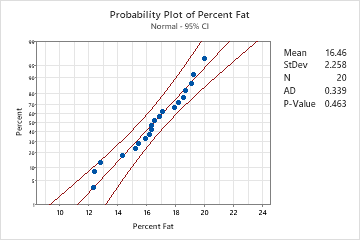# Example of Probability Plot

A scientist for a company that manufactures processed food wants to assess the percentage of fat in the company's bottled sauce. The advertised percentage is 15%. The scientist measures the percentage of fat in 20 random samples.

As part of the initial investigation, the scientist creates a probability plot to check for normality and to evaluate the distribution.

1. Open the sample data, FatContent.MTW.
2. Choose Graph > Probability Plot > Single.
3. In Graph variables, enter 'Percent Fat'.
4. Click OK.

## Interpret the results

The data points are relatively close to the fitted normal distribution line (the middle solid line of the graph). The p-value is greater than the significance level of 0.05. Therefore, the scientist fails to reject the null hypothesis that the data follow a normal distribution.

If you hold your pointer over the fitted distribution line of the graph in Minitab, a tooltip shows a table of percentiles and values. For the percent fat data, the tooltip indicates that 15% fat is at approximately the 30th percentile. Therefore, approximately 30% of the sauce has at least 15% fat or less, and 70% of the sauce has more than 15% fat.###### Note

For information on how to specify different distributions and parameters, go to Fitted distribution lines.

By using this site you agree to the use of cookies for analytics and personalized content.  Read our policy